CBSE (Science) Class 11CBSE
Share

# HC Verma solutions for Class 11 Physics chapter 14 - Some Mechanical Properties of Matter

#### HC Verma Concept of Physics Part-1 (2018-2019 Session) by H.C Verma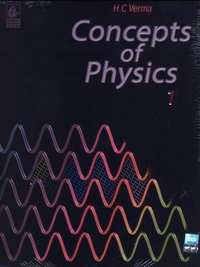## Chapter 14: Some Mechanical Properties of Matter

#### HC Verma solutions for Class 11 Physics Chapter 14 Exercise Short Answers [Pages 297 - 298]

Short Answers | Q 1 | Page 297

The ratio stress/strain remain constant for small deformation of a metal wire. When the deformation is made larger, will this ratio increase or decrease?

Short Answers | Q 2 | Page 297

When a block a mass M is suspended by a long wire of length L, the elastic potential potential energy stored in the wire is 1/2  × stress × strain × volume. Show that it is equal to 1/2  Mgl, where l is the extension. The loss in gravitational potential energy of the mass earth system is Mgl. Where does the remaining 1/2 Mgl energy go ?

Short Answers | Q 3 | Page 297

When the skeleton of an elephant and the skeleton of a mouse are prepared in the same size, the bones of the elephant are shown thicker than those of the mouse. Explain why the bones of an elephant are thicker than proportionate. The bones are expected to withstand the stress due to the weight of the animal.

Short Answers | Q 4 | Page 297

The yield point of a typical solid is about 1%. Suppose you are lying horizontally and two persons are pulling your hands and two persons are pulling your legs along your own length. How much will be the increase in your length if the strain is 1% ? Do you think your yield point is 1% or much less than that?

Short Answers | Q 5 | Page 297

When rubber sheets are used in a shock absorber, what happens to the energy of vibration?

Short Answers | Q 6 | Page 297

If a compressed spring is dissolved in acid, what happened to the elastic potential energy of the spring?

Short Answers | Q 7 | Page 297

A steel blade placed gently on the surface of water floats on it. If the same blade is kept well inside the water, it sinks. Explain.

Short Answers | Q 8 | Page 297

When some wax is rubbed on a cloth, it becomes waterproof. Explain.

Short Answers | Q 9 | Page 297

The contact angle between pure water and pure silver is 90°. If a capillary tube made of silver is dipped at one end in pure water, will the water rise in the capillary?

Short Answers | Q 10 | Page 297

It is said that a liquid rises or is depressed in capillary due to the surface tension. If a liquid neither rises nor depresses in a capillary, can we conclude that the surface tension of the liquid is zero?

Short Answers | Q 11 | Page 297

The contact angle between water and glass is 0°. When water is poured in a glass to the maximum of its capacity, the water surface is convex upward. The angle of contact in such a situation is more than 90°. Explain.

Short Answers | Q 12 | Page 297

A uniform vertical tube of circular cross section contains a liquid. The contact angle is 90°. Consider a diameter of the tube lying in the surface of the liquid. The surface to the right of this diameter pulls the surface on the left of it. What keeps the surface on the left in equilibrium?

Short Answers | Q 13 | Page 297

When a glass capillary tube is dipped at one end in water, water rises in the tube. The gravitational potential energy is thus increased. Is it a violation of conservation of energy?

Short Answers | Q 14 | Page 297

If a mosquito is dipped into water and released, it is not able to fly till it is dry again. Explain

Short Answers | Q 15 | Page 297

The force of surface tension acts tangentially to the surface whereas the force due to air pressure acts perpendicularly on the surface. How is then the force due to excess pressure inside a bubble balanced by the force due to the surface tension?

Short Answers | Q 16 | Page 297

When the size of a soap bubble is increased by pushing more air in it, the surface area increases. Does it mean that the average separation between the surface molecules is increased?

Short Answers | Q 17 | Page 298

Frictional force between solids operates even when they do not move with respect to each other. Do we have viscous force acting between two layers even if there is no relative motion?

Short Answers | Q 18 | Page 298

Water near the bed of a deep river is quiet while that near the surface flows. Give reasons.

Short Answers | Q 19 | Page 298

If water in one flask and castor oil in other are violently shaken and kept on a table, which will come to rest earlier?

#### HC Verma solutions for Class 11 Physics Chapter 14 Exercise MCQ, Short Answers [Pages 298 - 299]

MCQ | Q 1 | Page 298

A rope 1 cm in diameter breaks if the tension in it exceeds 500 N. The maximum tension that may be given to a similar rope of diameter 2 cm is

• 500 N

• 250 N

•  1000 N

• 2000 N

MCQ | Q 2 | Page 298

The breaking stress of a wire depends on

• material of the wire

•  length of the wire

• shape of he cross section.

MCQ | Q 3 | Page 298

A wire can sustain the weight of 20 kg before breaking. If the wire is cut into two equal parts, each part can sustain a weight of

• 10 kg

•  20 kg

•  40 kg

•  80 kg

MCQ | Q 4 | Page 298

Two wires A and B are made of same material. The wire A has a length l and diameter rwhile the wire B has a length 2l and diameter r/2. If the two wires are stretched by the same force, the elongation in A divided by the elongation in B is

• 1/8

• 1/4

• 4

• 8

MCQ | Q 5 | Page 298

A wire elongates by 1.0 mm when a load W is hung from it. If this wire goes over a a pulley and two weights W each are hung at the two ends, he elongation of he wire will be

•  0.5 m

• 1.0 mm

• 2.0 mm

• 4.0 mm

MCQ | Q 6 | Page 298

A heave uniform rod is hanging vertically form a fixed support. It is stretched by its won weight. The diameter of the rod is

•  smallest at the top and gradually increases down the rod

•  largest at the top and gradually decreased down the rod

• uniform everywhere

• maximum in the middle.

MCQ | Q 7 | Page 298

When a metal wire is stretched by a load, the fractional change in its volume ∆V/V is proportional to

• $\frac{∆ \text{l}}{\text{ l }}$

• $\left( \frac{∆ \text{ l }}{\text{ l }} \right)^2$

• $\sqrt{∆ \text{ l / l}}$

• none of these

MCQ | Q 8 | Page 298

The length of a metal wire is l1 when the tension in it T1 and is l2 when the tension is T2. The natural length of the wire is

• $\frac{\text{ l}_1 + \text{l}_2}{2}$

• $\sqrt{\text{ l}_1 \text{l}_2}$

• $\frac{\text{l}_1 \text{T}_2 - \text{l}_2 \text{T}_1}{\text{T}_2 - \text{T}_1}$

• $\frac{\text{l}_1 \text{T}_2 + \text{l}_2 \text{T}_1}{\text{T}_2 + \text{T}_1}$

MCQ | Q 9 | Page 298

A heavy mass is attached to a thin wire and is whirled in a vertical circle. The wire is most likely to break

•  when the mass is at the highest point

• when the mass is at the lowest point

• when the wire is horizontal

• at an angle of cos−1(1/3) from the upward vertical.

MCQ | Q 10 | Page 298

When a metal wire elongates by hanging a load on it, the gravitational potential energy is decreased.

•  This energy completely appears as the increased kinetic energy of the block.

• This energy completely appears as the increased elastic potential energy of the wire

•  This energy completely appears as heat.

• None of these.

MCQ | Q 11 | Page 298

By a surface of a liquid we mean

• a geometrical plane like x = 0

•  all molecules exposed to the atmosphere

•  a layer of thickness of the order of 10−8m

• a layer of thickness of the order of 10−4m

MCQ | Q 12 | Page 298

An ice cube is suspended in vacuum in a gravity free hall. As the ice melts it

•  will retain its cubical shape

• will change its shape to spherical

• will fall down on the floor of the hall

• will fly up.

MCQ | Q 13 | Page 298

When water droplets merge to form a bigger drop

•  energy is liberated

• energy is absorbed

• energy is neither liberated nor absorbed

• energy may either be liberated or absorbed depending on the nature of the liquid.

MCQ | Q 14 | Page 298

The dimension ML1T−2 can correspond to

• moment of a force

• surface tension

• modulus of elasticity

•  coefficient of viscosity

MCQ | Q 15 | Page 298

Air is pushed into a soap bubble of radius r to double its radius. If the surface tension of the soap solution in S, the work done in the process is

• 8 π r2 S

• 12 π r2 S

• 16 π r2 S

• 24 π r2 S

MCQ | Q 16 | Page 298

If more air is pushed in a soap bubble, the pressure in it

• decreases

•  increases

•  remains same

• becomes zero.

MCQ | Q 17 | Page 298

If two soap bubbles of different radii are connected by a tube,

• air flows from bigger bubble to the smaller bubble till the sizes become equal

•  air flows from bigger bubble to the smaller bubble till the sizes are interchanged

• air flows from the smaller bubble to the bigger

• there is no flow of air.

MCQ | Q 18 | Page 298

Figure shows a capillary tube of radius r dipped into water. If the atmospheric pressure is P0, the pressure at point A is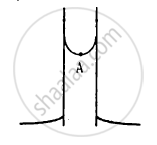• P0

• $P_0 + \frac{2S}{r}$

• $P_0 - \frac{2S}{r}$

• $P_0 - \frac{4S}{r}$

MCQ | Q 19 | Page 299

The excess pressure inside a soap bubble is twice the excess pressure inside a second soap bubble. The volume of the first bubble is n times the volume of the second where n is

• 4

• 2

• 1

• 0.125

Short Answers | Q 20 | Page 299

Which of the following graphs may represent the relation between the capillary rise hand the radius r of the capillary?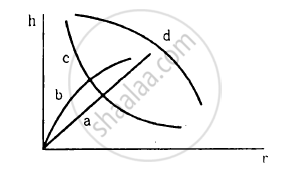MCQ | Q 21 | Page 299

Water rises in a vertical capillary tube up to a length of 10 cm. If the tube is inclined at 45°, the length of water risen in the tube will be

• 10 cm

• $10\sqrt{2}$ cm

• $10/\sqrt{2}$ cm

•  none of these

MCQ | Q 22 | Page 299

A 20 cm long capillary tube is dipped in water. The water rises up to 8 cm. If the entire arrangement is put in a freely falling elevator, the length of water column in the capillary tube will be

•  8 cm

•  6 cm

•  10 cm

•  20 cm

MCQ | Q 23 | Page 299

Viscosity is a property of

• liquids only

• solids only

• solids and liquids only

•  liquids and gases only.

MCQ | Q 24 | Page 299

The force of viscosity is

• electromagnetic

• gravitational

• nuclear

• weak

MCQ | Q 25 | Page 299

The viscous force acting between two layers of a liquid is given by $\frac{F}{A} = - \eta\frac{dv}{dz}$. This F/A may be called

•  pressure

•  longitudinal stress

•  tangential stress

• volume stress

MCQ | Q 26 | Page 299

A raindrop falls near the surface of the earth with almost uniform velocity because

• its weight is negligible

•  the force of surface tension balances its weight

•  the force of viscosity of air balance its weight

• the drops are charged and atmospheric electric field balances its weight.

MCQ | Q 27 | Page 299

A piece of wood is taken deep inside a long column of water and released. It will move up

•  with a constant upward acceleration

•  with a decreasing upward acceleration

•  with a deceleration

• with a uniform velocity

MCQ | Q 28 | Page 299

A solid sphere falls with a terminal velocity of 20 m s−1 in air. If it is allowed to fall in vacuum,

•  terminal velocity will be 20 m s−1

•  terminal velocity will be less than 20 m s−1

•  terminal velocity will be more than 20 m s−1

• there will be no terminal velocity

MCQ | Q 29 | Page 299

A spherical ball is dropped in a long column of a viscous liquid. The speed of the ball as a function of time may be best represented by the graph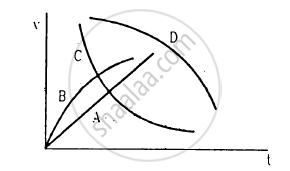• A

• B

• C

• D

#### HC Verma solutions for Class 11 Physics Chapter 14 Exercise MCQ [Page 299]

MCQ | Q 1 | Page 299

A student plots a graph from his reading on the determination of Young modulus of a metal wire but forgets to put the labels. the quantities on X and Y-axes may be respectively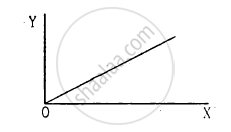(a) weight hung and length increased
(b) stress applied and length increased
(c) stress applied and strain developed
(d) length increased and the weight hung.

MCQ | Q 2 | Page 299

The properties of a surface are different from those of the bulk liquid because the surface molecules
(a) are smaller than other molecules
(b) acquire charge due to collision from air molecules
(c) find different type of molecules in their range of influence
(d) feel a net force in one direction.

MCQ | Q 3 | Page 299

The rise of a liquid in a capillary tube depends on

(a) the material
(b) the length
(d) the inner radius of the tube

MCQ | Q 4 | Page 299

The contact angle between a solid and a liquid is a property of

(a) the material of the solid
(b) the material of the liquid
(c) the shape of the solid
(d) the mass of the solid

MCQ | Q 5 | Page 299

A liquid is contained in a vertical tube of semicircular cross section. The contact angle is zero. The force of surface tension on the curved part and on the flat part are in ratio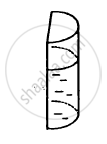• 1:1

•  1:2

• π:2

• 2:π

MCQ | Q 6 | Page 299

When a capillary tube is dipped into a liquid, the liquid neither rises nor falls in the capillary.
(a) The surface tension of the liquid must be zero.
(b) The contact angle must be 90°.
(c) The surface tension may be zero.
(d) The contact angle may be 90°.

MCQ | Q 7 | Page 299

A solid sphere moves at a terminal velocity of 20 m s−1 in air at a place where g = 9.8 m s−2. The sphere is taken in a gravity-free hall having air at the same pressure and  pushed down at a speed of 20 m s−1.

(a) Its initial acceleration will be 9.8 m s−2 downward.
(b) It initial acceleration will be 9.8 m s−2 upward.
(c) The magnitude of acceleration will decrease as the time passes.
(d) It will eventually stop

#### HC Verma solutions for Class 11 Physics Chapter 14 [Pages 300 - 302]

Q 1 | Page 300

A load of 10 kg is suspended by a metal wire 3 m long and having a cross-sectional area 4 mm2. Find (a) the stress (b) the strain and (c) the elongation. Young modulus of the metal is 2.0 × 1011 N m−2

Q 2 | Page 300

A vertical metal cylinder of radius 2 cm and length 2 m is fixed at the lower end and a load of 100 kg is put on it. Find (a) the stress (b) the strain and (c) the compression of the cylinder. Young modulus of the metal = 2 × 1011 N m−2.

Q 3 | Page 300

The elastic limit of steel is 8 × 108 N m−2 and its Young modulus 2 × 1011 N m−2. Find the maximum elongation of a half-metre steel wire that can be given without exceeding the elastic limit.

Q 4.1 | Page 300

A steel wire and a copper wire of equal length and equal cross-sectional area are joined end to end and the combination is subjected to a tension. Find the ratio of the stresses developed in the two wires .

Q 4.2 | Page 300

A steel wire and a copper wire of equal length and equal cross-sectional area are joined end to end and the combination is subjected to a tension. Find the ratio of the strains developed. Y of steel = 2 × 1011N m−2. Y of copper = 1.3 × 10 11 N m−2

Q 5 | Page 300

In figure the upper wire is made of steel and the lower of copper. The wires have equal cross section. Find the ratio of the longitudinal strains developed in the two wires.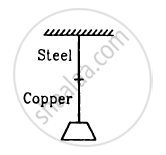Q 6.1 | Page 300

The two wires shown in figure are made of the same material which has a breaking stress of 8 × 108 N m−2. The area of cross section of the upper wire is 0.006 cm2 and that of the lower wire is 0.003 cm2. The mass m1 = 10 kg, m2 = 20 kg and the hanger is light.  Find the maximum load that can be put on the hanger without breaking a wire. Which wire will break first if the load is increased?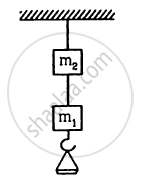Q 6.2 | Page 300

The two wires shown in figure are made of the same material which has a breaking stress of 8 × 108 N m−2. The area of cross section of the upper wire is 0.006 cm2 and that of the lower wire is 0.003 cm2. The mass m1 = 10 kg, m2 = 20 kg and the hanger is light.   Repeat the above part if m1 = 10 kg and m2 = 36 kg.Q 7 | Page 300

Two persons pull a rope towards themselves. Each person exerts a force of 100 N on the rope. Find the Young modulus of the material of the rope if it extends in length by 1 cm. Original length of the rope = 2 m and the area of cross section = 2 cm2

Q 8 | Page 300

A steel rod of cross-sectional area 4 cm2 and 2 m shrinks by 0.1 cm as the temperature decreases in night. If the rod is clamped at both ends during the day hours, find the tension developed in it during night hours. Young modulus of steel = 1.9 × 1011 N m−2.

Q 9 | Page 300

Consider the situation shown in figure. The force F is equal to the m2 g/2. If the area of cross section of the string is A and its Young modulus Y, find the strain developed in it. The string is light and there is no friction anywhere.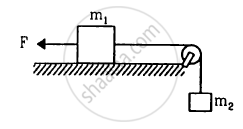Q 10 | Page 300

A sphere of mass 20 kg is suspended by a metal wire of unstretched length 4 m and diameter 1 mm. When in equilibrium, there is a clear gap of 2 mm between the sphere and the floor. The sphere is gently pushed aside so that the wire makes an angle θ with the vertical and is released. Find the maximum value of θ so that the sphere does not rub the floor. Young modulus of the metal of the wire is 2.0 × 1011 N m−2. Make appropriate approximations.

Q 11 | Page 301

A steel wire of original length 1 m and cross-sectional area 4.00 mm2 is clamped at the two ends so that it lies horizontally and without tensions. If a load of 2.16 kg is suspended from the middle point of the wire, what would be its vertical depression ? Y of the steel = 2.0 × 1011 N m−2. Take g = 10 m s−2

Q 12 | Page 301

A copper wire of cross-sectional area 0.01 cm2 is under a tension of 20N. Find the decrease in the cross-sectional area. Young modulus of copper = 1.1 × 1011 N m−2 and Poisson ratio = 0.32.

["Hint" : (Delta"A")/"A"=2(Delta"r")/"r"]

Q 13 | Page 301

Find the increase in pressure required to decrease the volume of a water sample by 0.01%. Bulk modulus of water = 2.1 × 109 N m−2.

Q 14 | Page 301

Estimate the change in the density of water in ocean at a depth of 400 m below the surface. The density of water at the surface = 1030 kg m−3 and the bulk modulus of water = 2 × 109 N m−2.

Q 15 | Page 301

A steel plate of face area 4 cm2 and thickness 0.5 cm is fixed rigidly at the lower surface. A tangential force of 10 N is applied on the upper surface. Find the lateral displacement of the upper surface with respect to the lower surface. Rigidity modulus of steel = 8.4 × 1010 N m−2

Q 16 | Page 301

A 5.0 cm long straight piece of thread is kept on the surface of water. Find the force with which the surface on one side of the thread pulls it. Surface tension of water = 0.076 N m−1.

Q 17 | Page 301

Find the excess pressure inside (a) a drop of mercury of radius 2 mm (b) a soap bubble of radius 4 mm and (c) an air bubble of radius 4 mm formed inside a tank of water. Surface tension of mercury, soap solution and water are 0.465 N m−1, 0.03 N m−1 and 0.076 N m−1 respectively.

Q 18 | Page 301

Consider a small surface area of 1 mm2 at the top of a mercury drop of radius 4.0 mm. Find the force exerted on this area (a) by the air above it (b) by the mercury below it and (c) by the mercury surface in contact with it. Atmospheric pressure = 1.0 × 105 Pa and surface tension of mercury = 0.465 N m−1.  Neglect the effect of gravity. Assume all numbers to be exact.

Q 19 | Page 301

The capillaries shown in figure have inner radii 0.5 mm, 1.0 mm and 1.5 mm respectively. The liquid in the beaker is water. Find the heights of water level in the capillaries. The surface tension of water is 7.5 × 10−2 N m−1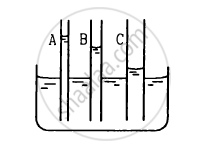Q 20 | Page 301

The lower end of a capillary tube is immersed in mercury. The level of mercury in the tube is found to be 2 cm below the outer level. If the same tube is immersed in water, up to what height will the water rise in the capillary?

Q 21 | Page 301

A barometer is constructed with its tube having radius 1.0 mm. Assume that the surface of mercury in the tube is spherical in shape. If the atmospheric pressure is equal to 76 cm of mercury, what will be the height raised in the barometer tube? The contact angle of mercury with glass = 135° and surface tension of mercury = 0.465 N m−1. Density of mercury = 13600 kg m−3

Q 22 | Page 301

A capillary tube of radius 0.50 mm is dipped vertically in a pot of water. Find the difference between the pressure of the water in the tube 5.0 cm below the surface and the atmospheric pressure. Surface tension of water = 0.075 N m−1.

Q 23 | Page 301

Find the surface energy of water kept in a cylindrical vessel of radius 6.0 cm. Surface tension of water = 0.075 J m−2.

Q 24 | Page 301

A drop of mercury of radius 2 mm is split into 8 identical droplets. Find the increase in surface energy. Surface tension of mercury = 0.465 J m−2.

Q 25 | Page 301

A capillary tube of radius 1 mm is kept vertical with the lower end in water. (a) Find the height of water raised in the capillary. (b) If the length of the capillary tube is half the answer of part , find the angle θ made by the water surface in the capillary with the wall.

Q 26 | Page 301

The lower end of a capillary tube of radius 1 mm is dipped vertically into mercury. (a) Find the depression of mercury column in the capillary. (b) If the length dipped inside is half the answer of part (a), find the angle made by the mercury surface at the end of the capillary with the vertical. Surface tension of mercury = 0.465 N m−1 and the contact angle of mercury with glass −135 °.

Q 27 | Page 301

Two large glass plates are placed vertically and parallel to each other inside a tank of water with separation between the plates equal to 1 mm. Find the rise of water in the space between the plates. Surface tension of water = 0.075 Nm−1.

Q 28 | Page 301

Consider an ice cube of edge 1.0 cm kept in a gravity-free hall. Find the surface area of the water when the ice melts. Neglect the difference in densities of ice and water.

Q 29 | Page 301

A wire forming a loop is dipped into soap solution and taken out so that a film of soap solution is formed. A loop of 6.28 cm long thread is gently put on the film and the film is pricked with a needle inside the loop. The thread loop takes the shape of a circle. Find the tension the the thread. Surface tension of soap solution = 0.030 N m−1.

Q 30 | Page 301

A metal sphere of radius 1 mm and mass 50 mg falls vertically in glycerine. Find (a) the viscous force exerted by the glycerine on the sphere when the speed of the sphere is 1 cm s1, (b) the hydrostatic force exerted by the glycerine on the sphere and (c) the terminal velocity with which the sphere will move down without acceleration. Density of glycerine = 1260 kg m−3 and its coefficient of viscosity at room temperature = 8.0 poise.

Q 31 | Page 301

Estimate the speed of vertically falling raindrops from the following data. Radius of the drops = 0.02 cm, viscosity of air = 1.8 × 10−4 poise, g= 9.9 × 10 ms2 and density of water = 1000 kg m−3.

Q 32 | Page 302

Water flows at a speed of 6 cm s1 through a tube of radius 1 cm. Coefficient of viscosity of water at room temperature is 0.01 poise. Calculate the Reynolds number. Is it a steady flow?

## Chapter 14: Some Mechanical Properties of Matter

#### HC Verma Concept of Physics Part-1 (2018-2019 Session) by H.C Verma## HC Verma solutions for Class 11 Physics chapter 14 - Some Mechanical Properties of Matter

HC Verma solutions for Class 11 Physics chapter 14 (Some Mechanical Properties of Matter) include all questions with solution and detail explanation. This will clear students doubts about any question and improve application skills while preparing for board exams. The detailed, step-by-step solutions will help you understand the concepts better and clear your confusions, if any. Shaalaa.com has the CBSE Concept of Physics Part-1 (2018-2019 Session) by H.C Verma solutions in a manner that help students grasp basic concepts better and faster.

Further, we at Shaalaa.com provide such solutions so that students can prepare for written exams. HC Verma textbook solutions can be a core help for self-study and acts as a perfect self-help guidance for students.

Concepts covered in Class 11 Physics chapter 14 Some Mechanical Properties of Matter are Introduction to Fluid Machanics, Archimedes' Principle, Stokes' Law, Equation of Continuity, Torricelli'S Law, Concept of Pressure, Pascal's Law, Variation of Pressure with Depth, Atmospheric Pressure and Gauge Pressure, Hydraulic Machines, STREAMLINE FLOW, Bernoulli’S Principle, Viscosity, Reynolds Number, Surface Tension, Effect of Gravity on Fluid Pressure, Terminal Velocity, Critical Velocity, Excess of Pressure Across a Curved Surface, Stress-strain Curve, Stress and Strain, Hooke’s Law, Young’s Modulus, Determination of Young’s Modulus of the Material of a Wire, Shear Modulus, Bulk Modulus, Applications of Elastic Behaviour of Materials, Elastic Energy, Elastic Behaviour of Solid.

Using HC Verma Class 11 solutions Some Mechanical Properties of Matter exercise by students are an easy way to prepare for the exams, as they involve solutions arranged chapter-wise also page wise. The questions involved in HC Verma Solutions are important questions that can be asked in the final exam. Maximum students of CBSE Class 11 prefer HC Verma Textbook Solutions to score more in exam.

Get the free view of chapter 14 Some Mechanical Properties of Matter Class 11 extra questions for Physics and can use Shaalaa.com to keep it handy for your exam preparation

S